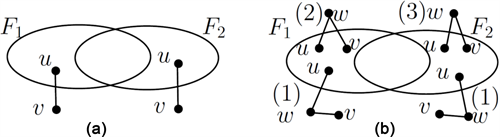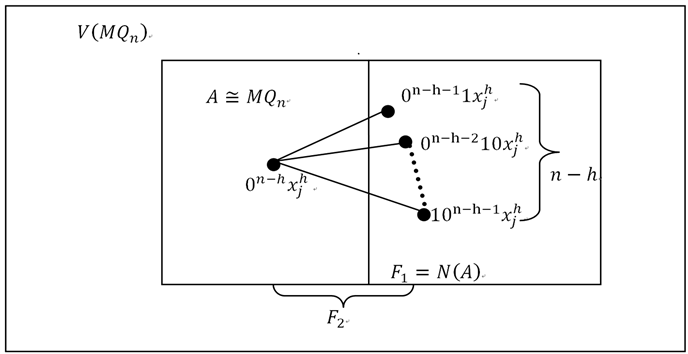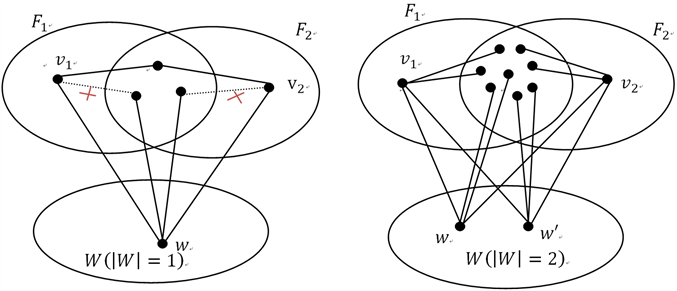# MO¨ bius立方体的h-好邻条件诊断度The h-Good-Neighbor Conditional Diagnosability of MO¨ bius Cubes

DOI: 10.12677/AAM.2018.71005, PDF, HTML, XML, 下载: 901  浏览: 1,807

Abstract: The conditional diagnosability is an important measure of the reliability of interconnection network. The “condition” means that all adjacent processors of any processor cannot be potentially faulty at the same time. As an improvement of conditional diagnosability, h-good-neighbor conditional diagnosability has been proposed by Peng et al. under the assumption that every fault-free node has at least h fault-free neighbors, which is an important indicator of reliability of interconnection network in the case of vertices failure. In this paper, we first introduced and investigated the h-good-neighbor conditional diagnosability of Möbius Cubes (MNQ). And we showed that the h-good- neighbor conditional diagnosability of Mo ̈bius Cubes is (n-h+1)2h-1(0≤h≤n-3) under the PMC and MM* model, which can be several times higher than the classical diagnosability of MNQ.

1. 引言Table 1. The test results under the PMC model and MM* model

2. 预备知识

2.1. 术语和概念

$H=\left({V}^{\prime },{E}^{\prime }\right)$$G=\left(V,E\right)$ ，如果 ${V}^{\prime }\subseteq V$${E}^{\prime }\subseteq E$ ，则称图H为G的子图，记为 $H\subseteq G$ 。若 ${V}^{\prime }=V$ ，此时则称子图H为G的支撑子图。对于图G中任意子集 ${V}^{\prime }\subseteq V\left(G\right)$ ，称以 ${V}^{\prime }$ 为点集，以图G中两端点均在 ${V}^{\prime }$ 的所有边为边集的子图为点集 ${V}^{\prime }$ 的导出子图，记作 $G\left[{V}^{\prime }\right]$ 。给定两个集合A，B，集合 $\left(A-B\right)\cup \left(B-A\right)$ 称为A与B的对称差，记为 $A\text{\hspace{0.17em}}\Delta \text{\hspace{0.17em}}B$ 。用 $G-A$ 表示从图G中删除顶点子集A所获得的子图，记为 $\stackrel{¯}{A}$ 。这里涉及未提到的术语可参考文献  。

DahBura与Masson  提出了一个多项式复杂度算法来检验一个系统是否是t-可诊断的。

Lai et al.  与Sengupta等人  分别提出了在PMC模型和MM*下故障集对 $\left({F}_{1},{F}_{2}\right)$ 可区分的充要条件。图1(a)，图1(b)分别给出了在PMC模型和MM*下可区分对 $\left({F}_{1},{F}_{2}\right)$ 的示例说明，即引理2.2和2.3。Figure 1. Illustration of a distinguishable pair $\left({F}_{1},{F}_{2}\right)$

1) 存在点 $u\in \left({F}_{1}\text{\hspace{0.17em}}\Delta \text{\hspace{0.17em}}{F}_{2}\right)$$v,w\in \stackrel{¯}{{F}_{1}\cup {F}_{2}}$ 满足 $uw,vw\in E\left(G\right)$

2) 存在点 $u,v\in \left({F}_{1}-{F}_{2}\right)$$w\in \stackrel{¯}{{F}_{1}\cup {F}_{2}}$ 满足 $uw,vw\in E\left(G\right)$

3) 存在点 $u,v\in \left({F}_{2}-{F}_{1}\right)$$w\in \stackrel{¯}{{F}_{1}\cup {F}_{2}}$ 满足 $uw,vw\in E\left(G\right)$

2.2. Möbius立方体网络

1995年，Cull和Larson  提出了n-维莫比乌斯(Möbius)立方体网络，记为 $M{Q}_{n}$ ，具有以下性质：(详见文献  )。这里用2元n序列来定义。

$V=\left\{{u}_{n-1}{u}_{n-2}\cdots {u}_{0}|{u}_{i}\in \left\{0,1\right\},i=0,2,\cdots ,n-1\right\}$

$u={u}_{n-1}{u}_{n-2}\cdots {u}_{0}\in V\left(M{Q}_{n}\right)$ 连接到另外n个点 ${u}^{i}\in V\left(M{Q}_{n}\right)$ ( $0\le i\le n-1$ )当且仅当：

${u}^{i}=\left\{\begin{array}{l}{u}_{n-1}{u}_{n-2}\cdots {u}_{i+1}\stackrel{¯}{{u}_{i}}{u}_{i-1}\cdots {u}_{0};\text{\hspace{0.17em}}\text{\hspace{0.17em}}{u}_{i+1}=0;\\ {u}_{n-1}{u}_{n-2}\cdots {u}_{i+1}\stackrel{¯}{{u}_{i}{u}_{i-1}\cdots {u}_{0}};\text{\hspace{0.17em}}\text{\hspace{0.17em}}\text{ }{u}_{i+1}=1.\end{array}$

3. Möbius立方体的h-好邻条件可诊断度Figure 2. Illustration of ${F}_{1}$ and ${F}_{2}$

$|{F}_{2}|=|{F}_{2}-{F}_{1}|+|{F}_{1}\cap {F}_{2}|\ge {2}^{h}+\left(n-h\right){2}^{h}$

$n\ge 5,0\le h\le n-3$ 时，因为 $V\left(M{Q}_{n}\right)={F}_{1}\cup {F}_{2}$ ，此时，

${2}^{n}=|V\left(M{Q}_{n}\right)|=|{F}_{1}\cup {F}_{2}|\le |{F}_{1}|+|{F}_{2}|\le 2\left({2}^{h}\left(n-h\right)+{2}^{h}-1\right)\le {2}^{n}-2$

$\forall w\in W$ ，有 ${N}_{M{Q}_{n}}\left(w\right)\subseteq {F}_{1}\cup {F}_{2}$ 。下面我们根据h的大小讨论以下两种情形来证明 $W=\varnothing$ 成立。

$\begin{array}{c}\underset{w\in W}{\sum }|{N}_{{F}_{1}\cap {F}_{2}}\left(w\right)|=|W|\left(n-2\right)\le \underset{v\in {F}_{1}\cap {F}_{2}}{\sum }{d}_{M{Q}_{n}}\left(v\right)\\ \le |{F}_{1}\cap {F}_{2}|n\le \left(|{F}_{2}|-1\right)n\le \left(2n-2\right)n\end{array}$

$\begin{array}{c}{2}^{n}=|V\left(M{Q}_{n}\right)|=|{F}_{1}\cup {F}_{2}|+|W|\le |{F}_{1}|+|{F}_{2}|+|W|\\ \le 2\left(2n-1\right)+2n+2<{2}^{n}\end{array}$

${N}_{{F}_{1}\cap {F}_{2}}\left(w\right)={d}_{M{Q}_{n}}\left(w\right)-\left\{{v}_{1},{v}_{2}\right\}$ 。由于 $V\left(M{Q}_{n}\right)$ 中任意两个顶点之间至多有两个公共邻点，因此，至多在W中存在两个孤立点。图3给出了W的两种情形。

$|W|=1$ ，不防设 $W=\left\{w\right\}$ ，则 $w$${v}_{1},{v}_{2}$ 是相邻的。由引理2.4知 $M{Q}_{n}$ 中无三角且任意两个顶点之间至多有两个公共邻点，故有 ${N}_{{F}_{1}\cap {F}_{2}}\left(w\right)\cap {N}_{{F}_{1}\cap {F}_{2}}\left({v}_{1}\right)=\varnothing$${N}_{{F}_{1}\cap {F}_{2}}\left(w\right)\cap {N}_{{F}_{1}\cap {F}_{2}}\left({v}_{2}\right)=\varnothing$${N}_{{F}_{1}\cap {F}_{2}}\left({v}_{1}\right)\cap {N}_{{F}_{1}\cap {F}_{2}}\left({v}_{2}\right)\le 1$ 。此时，当 $n\ge 4$ 时，

$\begin{array}{c}|{F}_{2}|=|{F}_{1}\cap {F}_{2}|+|{F}_{2}-{F}_{1}|\\ \ge |{N}_{{F}_{1}\cap {F}_{2}}\left(w\right)|+|{N}_{{F}_{1}\cap {F}_{2}}\left({v}_{1}\right)|+|{N}_{{F}_{1}\cap {F}_{2}}\left({v}_{2}\right)|-1+1\\ \ge n-2+n-1+n-1-1+1>2n-1\end{array}$

$|W|=2$ ，则 $N\left(\left\{{v}_{1},{v}_{2}\right\}\cup W\right)\subseteq {F}_{1}\cap {F}_{2}$ 。不妨设 $W=\left\{w,{w}^{\prime }\right\}$ ，则 ${w}^{\prime }$${v}_{1},{v}_{2}$ 是相邻的。因为 $M{Q}_{n}$ 中任意两个顶点之间至多有两个公共邻点，所以 ${v}_{1},{v}_{2},w,{w}^{\prime }$${F}_{1}\cap {F}_{2}$ 中无公共邻点。且当 $n\ge 4$ 时，

$2n-1\ge |{F}_{2}|=|{F}_{1}\cap {F}_{2}|+|{F}_{2}-{F}_{1}|\ge N\left(\left\{{v}_{1},{v}_{2}\right\}\cup W\right)+1\ge n-8+1>2n-1$

$W=\varnothing$$V\left(M{Q}_{n}\right)-{F}_{1}\cup {F}_{2}$ 中不存在孤立点，因此 $\left[V\left(M{Q}_{n}\right)-{F}_{1}\cup {F}_{2}\right]$ 中不存在边 $uv$ 使得 $u\left(v\right)$

${F}_{1}\text{\hspace{0.17em}}\Delta \text{\hspace{0.17em}}{F}_{2}$ 中的某些点是相邻的。否则， ${F}_{1}$${F}_{2}$ 是可区分的，与假设矛盾。因此，在 $V\left(M{Q}_{n}\right)-{F}_{1}\cup {F}_{2}$${F}_{1}\text{\hspace{0.17em}}\Delta \text{\hspace{0.17em}}{F}_{2}$ 之间不存在边。考虑到 ${F}_{1}$${F}_{2}$ 是h-好邻条件故障集，易知 ${F}_{1}\cap {F}_{2}$ 是h-好邻条件故障点割集且 $\delta \left(M{Q}_{n}\left[{F}_{2}-{F}_{1}\right]\right)\ge h$ ，结合引理2.5和2.6得， $|{F}_{2}-{F}_{1}|\ge {2}^{h}$$|{F}_{1}\cap {F}_{2}|\ge {\kappa }^{h}\left(M{Q}_{n}\right)=\left(n-h\right){2}^{h}$ 。此时，

$|{F}_{2}|=|{F}_{2}-{F}_{1}|+|{F}_{1}\cap {F}_{2}|\ge {2}^{h}+\left(n-h\right){2}^{h}$Figure 3. Illustration of the proof of $h=1$

4. 结论

  Preparata, F.P., Metze, G. and Chien, R.T. (2006) On the Connection Assignment Problem of Diagnosable Systems. IEEE Transactions on Electronic Computers, EC-16, 848-854. https://doi.org/10.1109/PGEC.1967.264748  Hakimi, S.L. and Amin, A.T. (1974) Characterization of Connection Assignment of Diagnosable Systems. IEEE Transactions on Computers, C-23, 86-88. https://doi.org/10.1109/T-C.1974.223782  Malek, M. (1980) A Comparison Connection Assignment for Diagnosis of Multiprocessor Systems. Symposium on Computer Architecture, La Baule, 6-8 May 1980, 31-36. https://doi.org/10.1145/800053.801906  Sengupta, A. and Dahbura, A.T. (1992) On Self-Diagnosable Multiprocessor Systems: Diagnosis by the Comparison Approach. IEEE Transactions on Computers, 41, 1386-1396. https://doi.org/10.1109/12.177309  Lai, P.L., Tan, J.J.M., Chang, C.P. and Hsu, L.H. (2005) Conditional Diagnosability Measures for Large Multiprocessor Systems. IEEE Transactions on Computers, 54, 165-175. https://doi.org/10.1109/TC.2005.19  Peng, S.-L., Lin, C.-K., Tan, J.J.M. and Hsu, L.-H. (2012) The g-Good-Neighbor Conditional Diagnosability of Hypercube under PMC Model. Applied Mathematics and Computation, 218, 10406-10412. https://doi.org/10.1016/j.amc.2012.03.092  Wang, S.Y. and Han, W.P. (2016) The g-Good-Neighbor Conditional Diagnosability of n-Dimensional Hypercubes under the MM* Model. Information Processing Letters, 116, 574-577. https://doi.org/10.1016/j.ipl.2016.04.005  Yuan, J., Liu, A.X., Ma, X., Liu, X.L., Qin, X. and Zhang, J.F. (2015) The g-Good-Neighbor Conditional Diagnosability of k-Ary n-Cubes under the PMC Model and MM* Model. IEEE Transactions on Parallel and Distributed Systems, 26, 1165-1177. https://doi.org/10.1109/TPDS.2014.2318305  Yuan, J., Liu, A.X., Qin, X., Zhang, J.F. and Li, J. (2016) g-Good-Neighbor Conditional Diagnosability Measure of 3-Ary n-Cube Networks. Theoretical Computer Science, 626, 144-162.  Lin, L., Xu, L., Wang, D. and Zhou, S. (2016) The g-Good-Neighbor Conditional Diagnosability of Arrangement Graphs. IEEE Transactions on Dependable and Secure Computing, PP, 1-12. https://doi.org/10.1109/TDSC.2016.2593446  徐俊明. 组合网络理论[M]. 北京: 科学出版社, 2007.  Dahbura, A.T. and Masson, G.M. (1984) An o(n^2.5) Fault Identiﬁcation Algorithm for Diagnosable Systems. IEEE Transactions on Computers, C-33, 486-492. https://doi.org/10.1109/TC.1984.1676472  Saad, Y. and Schultz, M.H. (1988) Topological Properties of Hypercubes. IEEE Transactions on Computers, 37, 867-872. https://doi.org/10.1109/12.2234  Cull, P. and Larson, S.M. (1995) The Mobius Cubes. IEEE Transactions on Computers, 44, 647-659. https://doi.org/10.1109/12.381950  Fan, J.X. (1998) Diagnosability of Möbius Cubes. IEEE Transactions on Parallel and Distributed Systems, 9, 923-928. https://doi.org/10.1109/71.722224  Li, X.J. and Xu, J.M. (2013) Edge-Fault Tolerance of Hypercube-Like Networks. Elsevier North-Holland, Inc. https://doi.org/10.1016/j.ipl.2013.07.010  Ye, L. and Liang, J. (2016) On Conditional h-Vertex Connectivity of Some Networks. Chinese Journal of Electronics, 25, 556-560. https://doi.org/10.1049/cje.2016.05.023